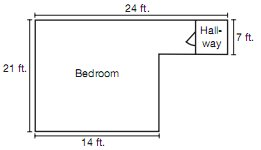# The Wrights plan to carpet their bedroom and the adjoining hallway as shown in the diagram. What is the area of the bedroom and hallway in square feet?Explanation

Think of the space as two rectangles: 21 ft. by 14 ft. and 10 ft. by 7 ft. (You can ﬁnd the length of the missing side of the smaller rectangle by subtracting: 24 − 14 = 10.) Use the formula A = lw to ﬁnd the area of each rectangle, and then combine to ﬁnd the total area of the ﬂoor to be carpeted:$$21 \times 14=294$$, and $$7 \times 10=70$$. Add the areas of the two rectangles: 294+70 = 364, so the Wrights will need to buy 364 square feet of carpeting.